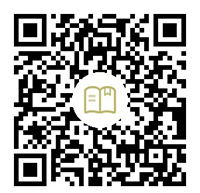# 迹忆客专注技术分享

## 在 Java 中从数字中获取 Unicode 字符

Unicode 是一种字符编码系统，它为编程语言中的每个字符和符号分配一个代码。由于没有其他编码标准涵盖所有语言，因此 Unicode 是唯一确保你可以使用任何语言组合检索或组合数据的编码方法。

Java 强烈支持 Unicode 字符。本教程将讨论如何从其编号创建 Unicode 字符。

## 在 Java 中使用强制转换获取 Unicode 字符

``````public class SimpleTesting {
public static void main(String args[]) {
int code = 0x2202;
System.out.println((char) code);
String code_str = Character.toString((char) code);
System.out.println(code_str);
}
}
``````

``````∂
∂
``````

`Character.toString()` 方法是一个重载方法，它将代码点作为参数并返回指定代码点的字符串表示形式。查看下面的代码以获取另一个示例。

``````public class SimpleTesting {
public static void main(String args[]) {
int code = 0x13434;
String code_str = Character.toString((char) code);
System.out.println(code_str);
}
}
``````

``````㐴
``````

## 在 Java 中使用 `String.valueOf()` 方法获取 Unicode 字符

``````public class SimpleTesting {
public static void main(String args[]) {
int code = 0x13434;
char ch_code = (char) code;
String code_str = String.valueOf(ch_code);
System.out.println(code_str);
}
}
``````

``````㐴
``````

``````public class SimpleTesting {
public static void main(String args[]) {
int code = 0x2202;
char ch_code = (char) code;
String code_str = String.valueOf(ch_code);
System.out.println(code_str);
}
}
``````

``````∂
``````

## 在 Java 中使用 `Character.toChars()` 方法获取 Unicode 字符

``````public class SimpleTesting {
public static void main(String args[]) {
String code = "2202";
String code_str = String.valueOf(Character.toChars(Integer.parseInt(code, 16)));
System.out.println(code_str);
}
}
``````

``````∂
``````

``````public class SimpleTesting {
public static void main(String args[]) {
String code = "1434";
String code_str = String.valueOf(Character.toChars(Integer.parseInt(code, 16)));
System.out.println(code_str);
}
}
``````

``````ᐴ
``````

## 相关文章

### 在 Java 中检查某个字符是否是数字## 热门标签